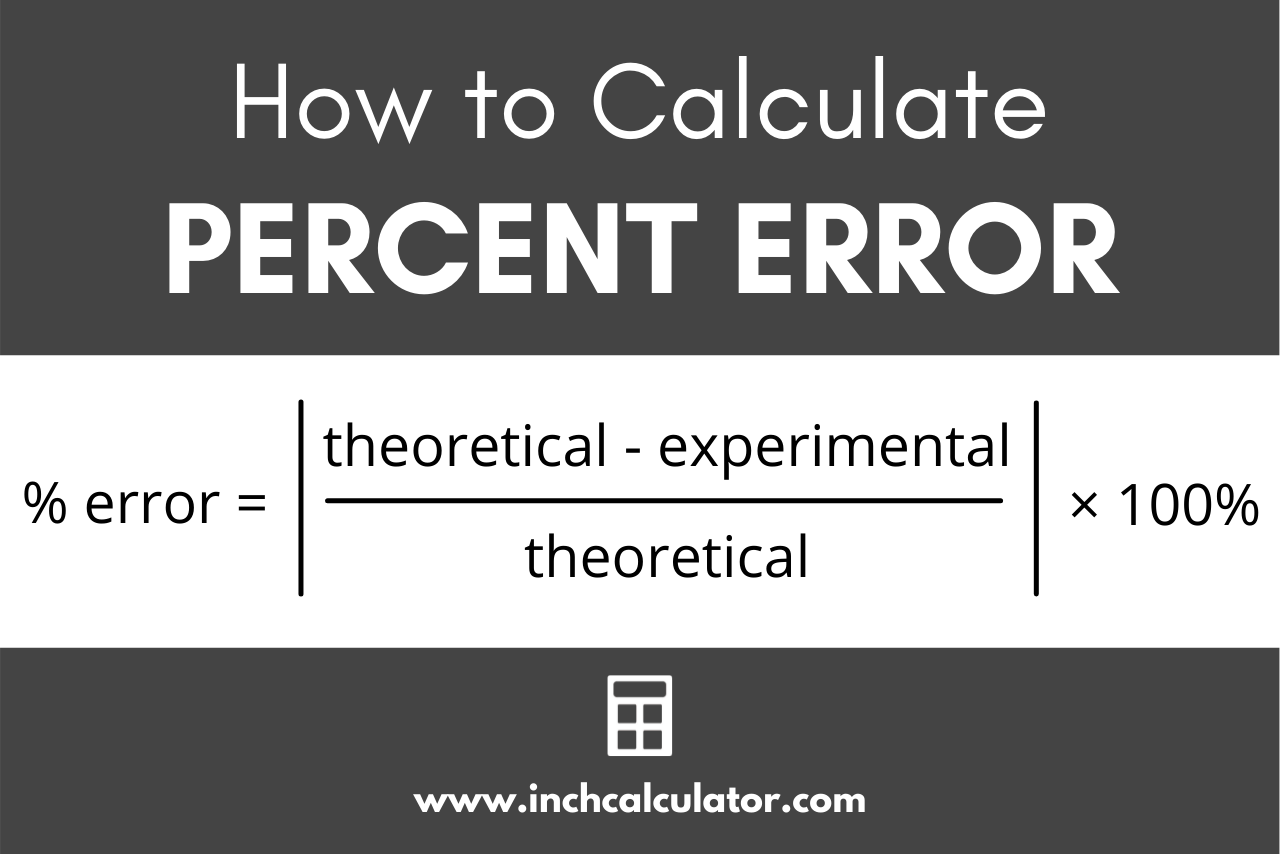# Percent Error Calculator

Calculate the percent error between a theoretical and experimental value, expressed as a percentage. The theoretical value is the expected value of measurement, while the experimental value is the actual value measured.

## Percent Error:

33.333%

### Steps to Solve Percent Error

% error=|theoretical - experimental| / |theoretical|×100
Substitute values in the equation
% error=|75 - 100| / |75|×100
Simplify and solve
% error=25 / 75×100%
% error=0.33333×100%
% error=33.333%
Learn how we calculated this below

## How to Calculate Percent Error

Percentage error is the margin of error in a measurement between the expected and actual values measured. It is used to express the difference between the expected values and actual values in an experiment as a percentage.

Measuring percent error is important because it illustrates how close a measured or actual value is to the expected, theoretical value in an experiment. In chemistry, it’s often used in tandem when measuring the percent yield of a reaction.

The expected value can also be called the theoretical, true, or accepted value. The actual value is sometimes referred to as the experimental value.### Percent Error Formula

You can find the percentage error using the following formula:

error = |theoretical – experimental| / |theoretical| × 100%

Thus, percent error equals the absolute value of the theoretical value minus the experimental value, divided by the theoretical value, multiplied by 100%.

To use the formula, first, find the difference by subtracting the experimental value from the theoretical value. Next, divide the difference by the theoretical value to find the relative error. Lastly, multiply by 100 to find the error as a percentage.

Our percent change calculator may also be useful for finding the amount of change between 2 values.

Example: Find the percent error between a theoretical value of 99 and an experimental value of 98.

% error = |theoretical – experimental| / |theoretical| × 100%
% error = |99 – 98| / |99| × 100%
% error = 1 / 99 × 100%
% error = .010101 × 100%
% error = 1.01%

### What is Percent Error

Since it is impossible to avoid measurement errors completely, there needs to be a way to define how much error there is when measuring.

Percent error is a measurement of the relative error between measurements in an experiment. You can find the precision, accuracy, or size of the error between measurements in an experiment from the percent error.

### Error Terminology

When conducting an experiment, the theoretical value is often referred to as the true value. This is the value expected to be seen when conducting a measurement.

The experimental value can also be called the observed value and is the value measured when conducting a measurement.

You might have also heard the term absolute error. Be mindful that absolute error and percent error are not the same thing.

Absolute error is the difference between the theoretical and experimental values.

absolute error = theoretical – experimental

Relative error is the difference between the theoretical and experimental values, divided by the theoretical value. Relative error is thus the amount of error relative to the whole.

relative error = theoretical – experimental / theoretical

Standard error is a statistical measure of error based on the standard deviation of the results. You can use our standard error calculator to find it.

Now you can solve percentage error using the formula above or our handy calculator. Use our percentage calculator for more tools to calculate percentages.

### Is percent error the same as percent difference?

No, percent error is the margin of error in a measurement between the expected and actual values measured, while percent difference uses true values.

### How is the percent error different from the margin of error?

The percent error is an absolute value, whereas the margin of error is a range of values.

### When should you use percent error?

You should use percent error when trying to compare measured versus known values.

### Can percent error be negative?

Yes, if the observed value is smaller than the true value, the percent error will be negative.

## References

1. Wu, G., Baraldo, M., Furlanut, M., Calculating percentage prediction error: A user's note,
Pharmacological Research
, October 1995, 32(4), 241-248. https://doi.org/10.1016/S1043-6618(05)80029-5